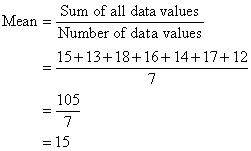# Mean Java Program In 4 Simple Methods | Java Programs

Java Program To Calculate Mean – In this article, we will brief in the various methods to calculate the mean in Java Programming. For mean java source code, we have written in multiple ways. If you have any queries let us know in comments.

• Using Standard Method
• Using Scanner Class
• Using Command Line Arguments
• Using Static Method
• Using Separate Class

Mean is one of the most used operations in the field of probability and statistics. In a given number of values, mean is the value that is the average of all of those.

Also check:You can see in the image uploaded above, the mean of the given values is basically the average of all.

Mean = (15 +13 + 18 + 16 + 14 + 17 + 12) / 7 = 105 / 7 = 15.

Thus, the various methods used to calculate the mean in Java Programming are as follows:

## Mean Java Program

In the standard method, the input values that are predetermined are given in the code. To find the mean of all the elements, the first thing required is an array of fixed length that is used to store all the values

Mean=Sum of all elements/total number of elements

So, to find the mean, we need to first traverse through the array and add up all the elements and store this value in another variable named sum until we reach the end of array.

Output:

Using Scanner Class

A code is written to generalize for different use-case or test-case and not just for a single input value. Therefore, predetermining and fixing the values beforehand in the code itself is not an appropriate way to write the code.

To overcome this scenario, we can make use of a special class named the Scanner Class in Java.

This class allows us to read inputs at runtime by the user. This can be used for any primitive data types.

So in this problem as well, we can make use of scanner class to read the length of the array first and then read the elements of the array based on the length given earlier.

After reading the input elements, the steps to perform and the logic is the same, first we calculate the sum of all the elements using a loop to traverse until the end of array and then divide this sum by the length of the array.

Output

### Calculate Mean – Using Command Line Arguments

Command line arguments can also be used as one of the ways to test the code using input convenient for us at runtime.

In here, during the process of writing the command for running the code itself we give inputs with space in between.

After writing the name of the code followed by space, we first give the number of elements to be stored in the array or in short the size of the array.

After giving the size, then those many data values are giving following space between each of them.

This is our input which is to be used for the rest of the program execution. But before making use of these inputs, these have to be converted to their desired types be it int or double.

After assigning the input values and storing it in respective variables, we then perform the same operations as used above.

Output:

Using Static Method

Writing the entire program with logic in a single method could be confusing when one would like to refer to the code later and make changes.

To avoid this situation, it is always safe to split up the code and write in different method blocks.

Hence, another static method can be made use of for this problem as well.

The main method could consist of the input/output part while the logic and operations to be implemented can be written in another static block.

This method will consist of statements to perform the sum of all the data values in the array followed by deriving mean from it by dividing the sum by the length of array (total number of data values).

Output:

Using Separate Class

It is always observed that, writing code in separate classes increases the readability of the code and makes it efficient to handle the code. It also helps in enhancing the security because, no two classes will we aware of the data or information the other class will contain.

x

## Plus Star Pattern Java Program | Patterns

Java program to print plus star pattern program – We have written the below print/draw ...